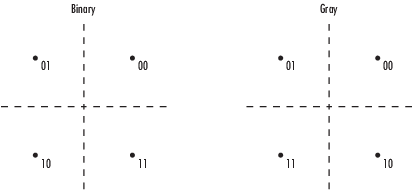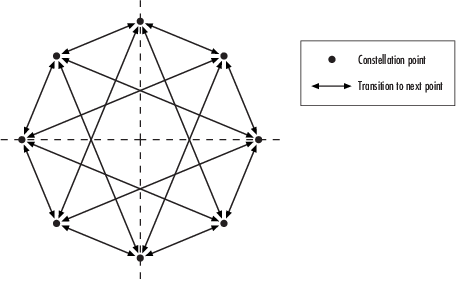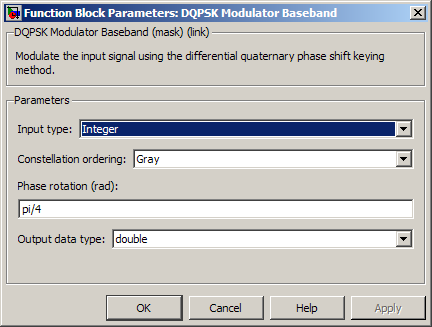DQPSK Modulator Baseband

Modulate using differential quadrature phase shift keying method

Library

PM, in Digital Baseband sublibrary of Modulation

•Description

The DQPSK Modulator Baseband block modulates using the differential quadrature phase shift keying method. The output is a baseband representation of the modulated signal.

The input must be a discrete-time signal. For information about the data types each block port supports, see Supported Data Types.

Integer-Valued Signals and Binary-Valued Signals

When you set the Input type parameter to Integer, the valid input values are 0, 1, 2, and 3. In this case, the block accepts a scalar or column vector input signal. If the first input is m, then the modulated symbol is

exp(jθ + jπm/2)

where θ represents the Phase rotation parameter. If a successive input is m, then the modulated symbol is the previous modulated symbol multiplied by exp(jθ + jπm/2).

When you set the Input type parameter to Bit, the input contains pairs of binary values. In this case, the block accepts a column vector whose length is an even integer. The following figure shows the complex numbers by which the block multiples the previous symbol to compute the current symbol, depending on whether you set the Constellation ordering parameter to Binary or Gray. The following figure assumes that you set the Phase rotation parameter to $\frac{\Pi }{4}$; in other cases, the two schematics would be rotated accordingly.The following figure shows the signal constellation for the DQPSK modulation method when you set the Phase rotation parameter to $\frac{\Pi }{4}$. The arrows indicate the four possible transitions from each symbol to the next symbol. The Binary and Gray options determine which transition is associated with each pair of input values.More generally, if the Phase rotation parameter has the form $\frac{\Pi }{k}$for some integer k, then the signal constellation has 2k points.

Dialog BoxInput type

Indicates whether the input consists of integers or pairs of bits.

Constellation ordering

Determines how the block maps each pair of input bits to a corresponding integer, using either a Binary or Gray mapping scheme.

The phase difference between the previous and current modulated symbols when the input is zero.

Output Data type

The output data type can be either single or double. By default, the block sets this to double.

Supported Data Types

PortSupported Data Types

Input

• Double-precision floating point

• Single-precision floating point

• Boolean when Input type is Bit

• 8-, 16-, and 32-bit signed integers

• 8-, 16-, and 32-bit unsigned integers

Output

• Double-precision floating point

• Single-precision floating point

Pair Block

DQPSK Demodulator Baseband

Extended Capabilities

C/C++ Code GenerationGenerate C and C++ code using Simulink® Coder™.

Introduced before R2006a